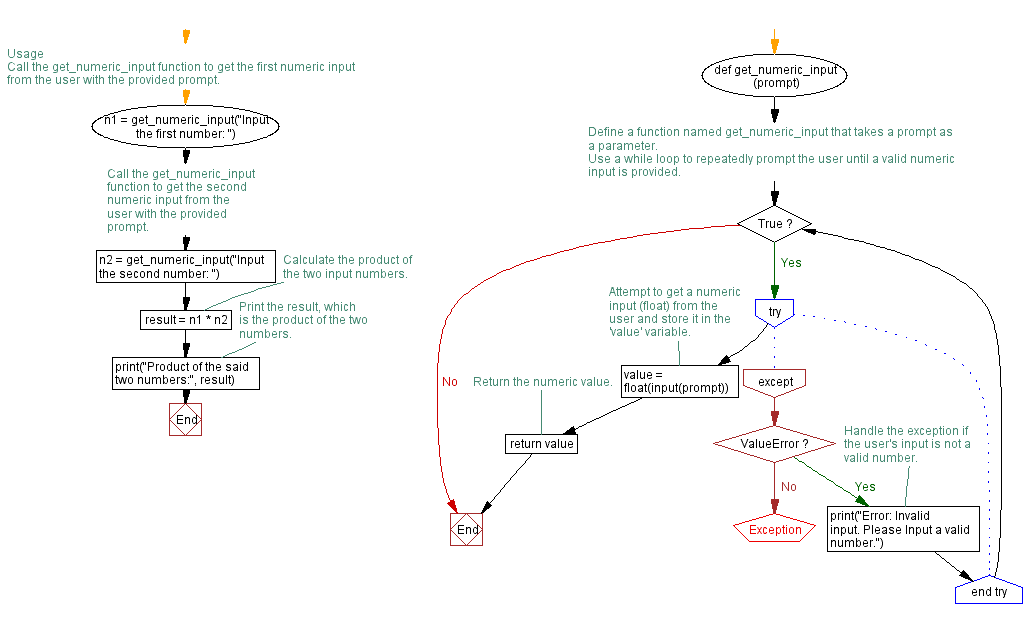﻿ Python program for valid numeric input

# Handling TypeError Exception in Python numeric input program

## Python Exception Handling: Exercise-4 with Solution

Write a Python program that prompts the user to input two numbers and raises a TypeError exception if the inputs are not numerical.

exception TypeError:

Raised when an operation or function is applied to an object of inappropriate type. The associated value is a string giving details about the type mismatch.

Sample Solution:

Code:

``````# Define a function named get_numeric_input that takes a prompt as a parameter.
def get_numeric_input(prompt):
# Use a while loop to repeatedly prompt the user until a valid numeric input is provided.
while True:
try:
# Attempt to get a numeric input (float) from the user and store it in the 'value' variable.
value = float(input(prompt))
# Return the numeric value.
return value
except ValueError:
# Handle the exception if the user's input is not a valid number.
print("Error: Invalid input. Please Input a valid number.")

# Usage
# Call the get_numeric_input function to get the first numeric input from the user with the provided prompt.
n1 = get_numeric_input("Input the first number: ")
# Call the get_numeric_input function to get the second numeric input from the user with the provided prompt.
n2 = get_numeric_input("Input the second number: ")
# Calculate the product of the two input numbers.
result = n1 * n2
# Print the result, which is the product of the two numbers.
print("Product of the said two numbers:", result)
``````

Explanation:

In the above code, the "get_numeric_input()" function takes a prompt parameter representing the message displayed to the user for input. Inside the function, a while loop continuously prompts the user for input until a valid number is entered.

Within the try block, we use the float() function to convert the user input to a float. We return the floating value if the conversion succeeds.

The program jumps to the except block if the conversion fails and a ValueError exception is raised. In the except block, we catch the ValueError exception and print an error message indicating the input is invalid. The loop continues, prompting the user for input again.

In the example usage section, we call the "get_numeric_input()" function twice to obtain two numerical inputs from the user. The inputs are stored in n1 and n2 respectively. We then perform a simple multiplication on the numbers and print the result.

Output:

```Input the first number: a
Error: Invalid input. Please Input a valid number.
Input the first number: 10
Input the second number: b
Error: Invalid input. Please Input a valid number.
Input the second number: 12
Product of the said two numbers: 120.0
```

Flowchart:What is the difficulty level of this exercise?

Test your Programming skills with w3resource's quiz.

﻿